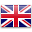# Mathematics

At St Kevin’s students learn to appreciate mathematics as an essential and relevant part of life by developing their ability to work mathematically.

The Mathematics K – 10 Syllabus is organised into four strands – one process strand, Working Mathematically, and three content strands: Number and Algebra, Statistics and Probability, Measurement and Geometry. There are sixteen sub-strands branching out from the three content strands. Working Mathematically encompasses processes that are embedded into the other strands through the content.

Our aim is for our students to:
• be confident, creative users and communicators of mathematics
• be able to investigate, represent and interpret situations in the world around them
• develop an increasingly sophisticated understanding of mathematical concepts and fluency with mathematical processes
• be able to pose and solve problems and reason in Number and Algebra, Measurement and Geometry, and Statistics and Probability
• recognise connections between the areas of mathematics and other disciplines and appreciate mathematics as an accessible, enjoyable discipline to study, and an important aspect of lifelong learning.
View the content of the NSW Mathematics K-10 Syllabus for the Australian curriculum.English